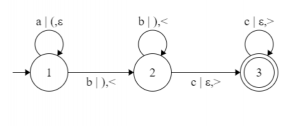Theory of Computation | L-graphs and what they represent

Prerequisite – Finite automata introduction
All programming languages can be represented as a finite automata. C, Paskal, Haskell, C++, all of them have a specific structure, grammar, that can be represented by a simple graph. Most of the graphs are NFA’s or DFA’s. But NFA’s and DFA’s determine the simplest possible language group: group of regular languages [Chomsky’s hierarchy]. This leaves us with a question: what about all other types of languages? One of the answers is Turing machine, but a Turing machine is hard to visualize. This is why in this article I will tell you about a type of finite automata called an L-graph.

In order to understand how L-graphs work we need to know what type of languages L-graphs determine. To put it simply, L-graphs represent context-sensitive type of languages [and every other type that the context-sensitive group contains]. If you don’t know what “context-sensitive” means, let me show you an example of a language that can be represented by an L-graph and not by any easier type of finite automata.

This language is. Corresponding L-graph looks like this:As you can see the brackets after the symbol ‘|’ control the numbers of symbols that come after the symbols ‘a’. This leads us to the two features that all L-graphs possess: all L-graphs have up to two independent from each other and from input symbols bracket groups, both bracket groups have to be right [string from a Dyck language] in order for the string of input symbols to be accepted by the given L-graph.

You can see that an L-graph is just a version of finite automata with an added couple of bracket groups. To help you get an understanding of why the languages determined by L-graphs are context-sensitive, check what strings the L-graph shown above has to accept.To conclude, I would like to add three other definitions that I’ll be using in the future. These definitions are very important for the hypothesis [and its future proof or disproof]. Refer – Hypothesis (language regularity) and algorithm (L-graph to NFA)

We will call a path in the L-graph neutral, if both bracket strings are right. If a neutral path T can be represented like this, T =, whereandare cycles andis a neutral path (,orcan be empty), T is called a nest. We can also say that the three (,,) is a nest or thatandform a nest in the path T.

(, d)-core in an L-graph G, defined as Core(G,, d), is a set of (, d)-canons. (, d)-canon, whereand d are positive whole numbers, is a path that contains at most m,, neutral cycles and at most k, kd, nests that can be represented this way:is part of the path T,, i = 1 or 3,, are cycles, every pathis a nest, where=,.

The last definition is about a context free L-graph. An L-graph G is called context free if G has only one bracket group (all rules in the L-graph have only one look of these two: [‘symbol’ | ‘bracket’, ?] or [‘symbol’ | ?, ‘bracket’]).

[Definition of a Dyck language.andare disjoint alphabets. There exists a bijection (function that for every element from the 1st set matches one and only one element from the 2nd set). Then the language defined by the grammar,, we will call a Dyck language.]

My Personal Notes arrow_drop_upCheck out this Author's contributed articles.

If you like GeeksforGeeks and would like to contribute, you can also write an article using contribute.geeksforgeeks.org or mail your article to contribute@geeksforgeeks.org. See your article appearing on the GeeksforGeeks main page and help other Geeks.

Please Improve this article if you find anything incorrect by clicking on the "Improve Article" button below.

Article Tags :

1

Please write to us at contribute@geeksforgeeks.org to report any issue with the above content.